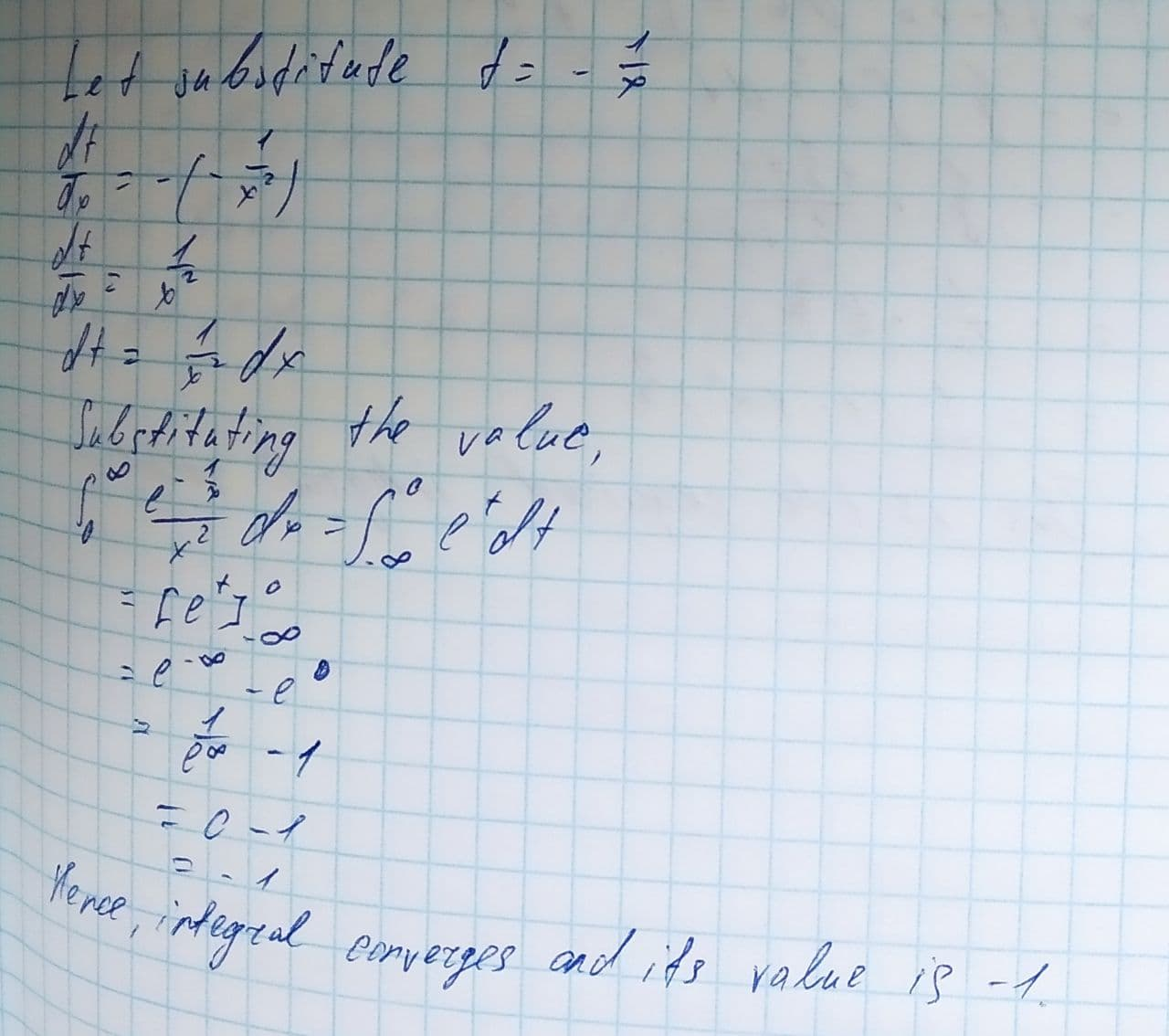Question# Determine whether the improper integral diverges or converges. Evaluate the integral if it converges: \int_{0}^{\infty}\frac{e^{\frac{-1}{x}}}{x^{2}}dx

Applications of integrals
ANSWEREDDetermine whether the improper integral diverges or converges. Evaluate the integral if it converges.
$$\displaystyle{\int_{{{0}}}^{{\infty}}}{\frac{{{e}^{{{\frac{{-{1}}}{{{x}}}}}}}}{{{x}^{{{2}}}}}}{\left.{d}{x}\right.}$$